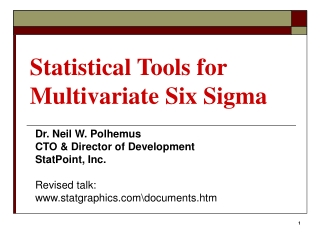# Statistical Tools for Multivariate Six Sigma - PowerPoint PPT PresentationDownload PresentationStatistical Tools for Multivariate Six Sigma

Statistical Tools for Multivariate Six Sigma
Download Presentation## Statistical Tools for Multivariate Six Sigma

- - - - - - - - - - - - - - - - - - - - - - - - - - - E N D - - - - - - - - - - - - - - - - - - - - - - - - - - -
##### Presentation Transcript

1. Statistical Tools for Multivariate Six Sigma Dr. Neil W. Polhemus CTO & Director of Development StatPoint, Inc. Revised talk: www.statgraphics.com\documents.htm

2. The Challenge The quality of an item or service usually depends on more than one characteristic. When the characteristics are not independent, considering each characteristic separately can give a misleading estimate of overall performance.

3. The Solution Proper analysis of data from such processes requires the use of multivariate statistical techniques.

4. Important Tools • Statistical Process Control • Multivariate capability analysis • Multivariate control charts • Statistical Model Building* • Data Mining - dimensionality reduction • DOE - multivariate optimization * Regression and classification.

5. Example #1 Textile fiber Characteristic #1: tensile strength (115.0 ± 1.0) Characteristic #2: diameter (1.05 ± 0.01)

6. Individuals Charts

7. Capability Analysis (each separately)

8. Scatterplot

9. Multivariate Normal Distribution

10. Control Ellipse

11. Multivariate Capability Determines joint probability of being within the specification limits on all characteristics.

12. Mult. Capability Indices Defined to give the same DPM as in the univariate case.

13. More than 2 Variables

14. Hotelling’s T-Squared Measures the distance of each point from the centroid of the data (or the assumed distribution).

15. T-Squared Chart

16. T-Squared Decomposition

17. Statistical Model Building • Defining relationships (regression and ANOVA) • Classifying items • Detecting unusual events • Optimizing processes When the response variables are correlated, it is important to consider the responses together. When the number of variables is large, the dimensionality of the problem often makes it difficult to determine the underlying relationships.

18. Example #2

19. Matrix Plot

20. Multiple Regression

21. Reduced Models MPG City = 29.9911 - 0.0103886*Weight + 0.233751*Wheelbase (R2=73.0%) MPG City = 64.1402 - 0.054462*Horsepower - 1.56144*Passengers - 0.374767*Width (R2=64.3%)

22. Dimensionality Reduction Construction of linear combinations of the variables can often provide important insights. • Principal components analysis (PCA) and principal components regression (PCR): constructs linear combinations of the predictor variables X that contain the greatest variance and then uses those to predict the responses. • Partial least squares (PLS): finds components that minimize the variance in both the X’s and the Y’s simultaneously.

23. Principal Components Analysis

24. Scree Plot

25. Component Weights C1 = 0.377*Engine Size + 0.292*Horsepower + 0.239*Passengers + 0.370*Length + 0.375*Wheelbase + 0.389*Width + 0.360*U Turn Space + 0.396*Weight C2 = -0.205*Engine Size – 0.593*Horsepower + 0.731*Passengers + 0.043*Length + 0.260*Wheelbase – 0.042*Width – 0.026*U Turn Space – 0.030*Weight

26. Interpretation

27. PC Regression

28. Contour Plot

29. PLS Model Selection

30. PLS Coefficients Selecting to extract 3 components:

31. Interpretation

32. Neural Networks

33. Bayesian Classifier

34. Classification

35. Design of Experiments When more than one characteristic is important, finding the optimal operating conditions usually requires a tradeoff of one characteristic for another. One approach to finding a single solution is to use desirability functions.

36. Example #3 Myers and Montgomery (2002) describe an experiment on a chemical process (20-run central composite design):

37. Optimize Conversion

38. Optimize Activity

39. Desirability Functions • Maximization

40. Desirability Functions • Hit a target

41. Combined Desirability di = desirability of i-th response given the settings of the m experimental factors X. D ranges from 0 (least desirable) to 1 (most desirable).

42. Desirability Contours Max D=0.959 at time=11.14, temperature=210.0, and catalyst = 2.20.

43. Desirability Surface

44. References • Johnson, R.A. and Wichern, D.W. (2002). Applied Multivariate Statistical Analysis. Upper Saddle River: Prentice Hall.Mason, R.L. and Young, J.C. (2002). • Mason and Young (2002). Multivariate Statistical Process Control with Industrial Applications. Philadelphia: SIAM. • Montgomery, D. C. (2005). Introduction to Statistical Quality Control, 5th edition. New York: John Wiley and Sons. • Myers, R. H. and Montgomery, D. C. (2002). Response Surface Methodology: Process and Product Optimization Using Designed Experiments, 2nd edition. New York: John Wiley and Sons. Revised talk: www.statgraphics.com\documents.htm